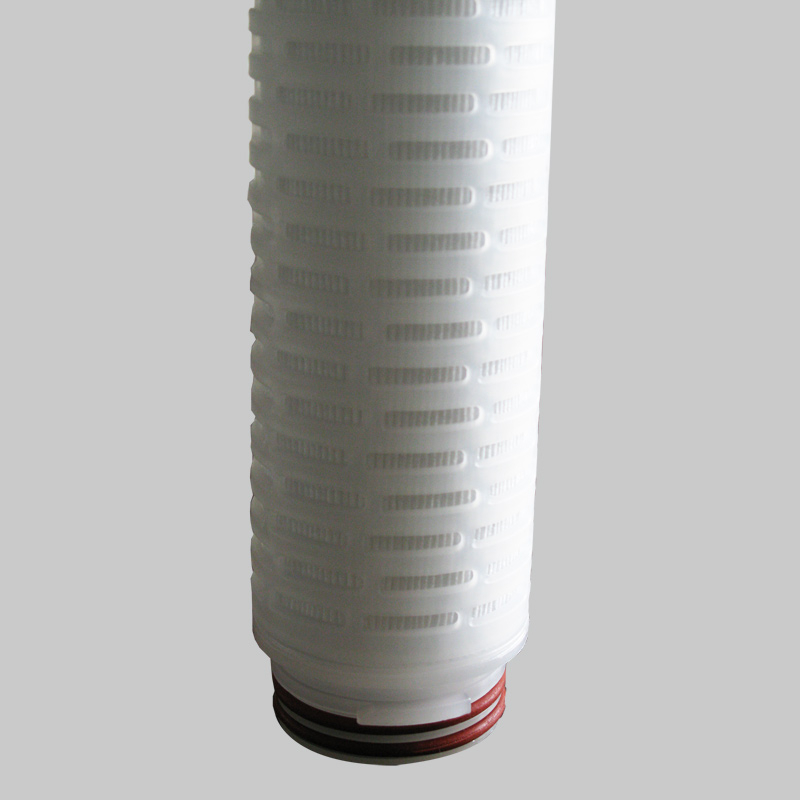20年专注过滤设备：微孔膜滤芯大流量滤芯过滤器 —— 做诚信品牌，赢精彩未来！
全国服务热线：0311-66685291

## 推荐产品# 玻璃纤维空气滤芯

YTBX系列滤芯采用复合玻璃纤维滤膜，以长纤复合无纺布作保护层，防止有脱落造成二次污染。

• 0311-66685291

YTBX系列玻璃纤维气体滤芯
YTBX系列滤芯采用复合玻璃纤维滤膜，以长纤复合无纺布作保护层，防止有脱落造成二次污染。

 滤芯结构 过滤面积 ≥0.6㎡ 过滤膜 玻璃纤维 导流层 进口无纺布 内支撑、端盖、外壳 聚丙烯、不锈钢 密封圈 硅橡胶、氟橡胶、三元乙丙、包氟 ≤90℃ 工作条件 工作温度 工作压差 0.4Mpa/23℃

 过滤精度 长度 接口形式 密封圈材质 YTBX 0030=0.3um 05=5英寸 2=222 G=硅橡胶 0050=0.5um 10=10英寸 6=226 S=三元乙丙 0100=1um 20=20英寸 15=215 P=包氟 0300=3um 30=30英寸 P=平头带垫片 F=氟橡胶 0500=5um 40=40英寸 2G=222衬钢 1000=10um 6G=226衬钢YTBX系列玻璃纤维气体滤芯
YTBX系列滤芯采用复合玻璃纤维滤膜，以长纤复合无纺布作保护层，防止有脱落造成二次污染。

 滤芯结构 过滤面积 ≥0.6㎡ 过滤膜 玻璃纤维 导流层 进口无纺布 内支撑、端盖、外壳 聚丙烯、不锈钢 密封圈 硅橡胶、氟橡胶、三元乙丙、包氟 ≤90℃ 工作条件 工作温度 工作压差 0.4Mpa/23℃

 过滤精度 长度 接口形式 密封圈材质 YTBX 0030=0.3um 05=5英寸 2=222 G=硅橡胶 0050=0.5um 10=10英寸 6=226 S=三元乙丙 0100=1um 20=20英寸 15=215 P=包氟 0300=3um 30=30英寸 P=平头带垫片 F=氟橡胶 0500=5um 40=40英寸 2G=222衬钢 1000=10um 6G=226衬钢## 联系我们

地址 ：石家庄市天山大街266号方大科技园 5号楼5层

手机：17731135566 / 18631187252

E-mail:
apdragon@163.com

## 产品分类

地址 ：石家庄市天山大街266号方大科技园 5号楼5层

手机：17731135566 / 18631187252

E-mail:
apdragon@163.com

## 官网二维码冀ICP备2020030002号   技术支持：【融创传媒